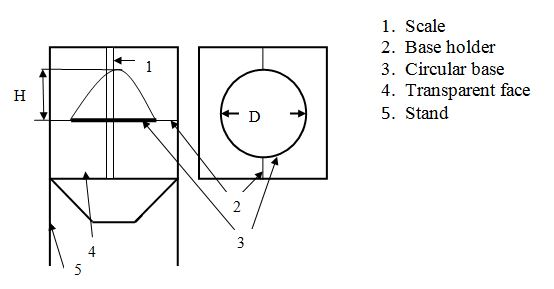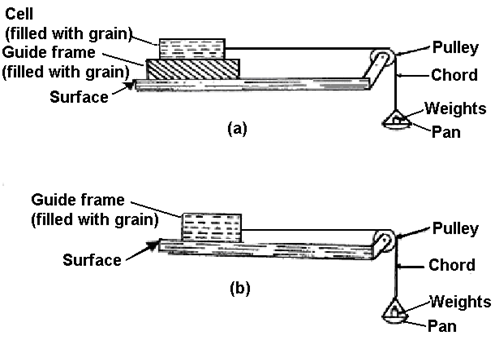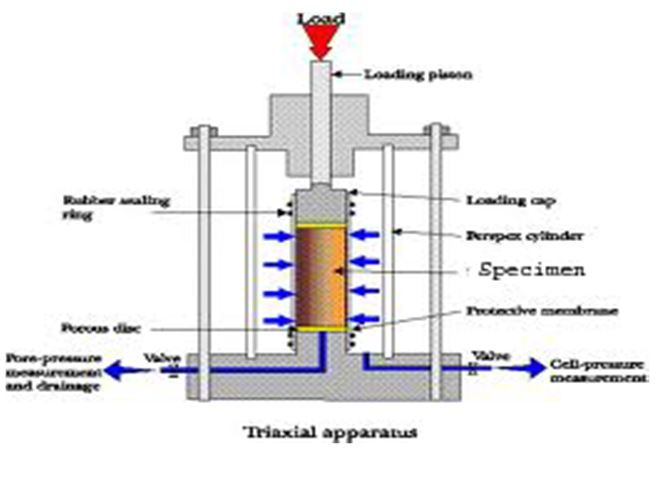## Lesson 8. Frictional properties of biomaterials

Frictional properties such as angle of repose and coefficient of friction are important in designing equipment for solid flow and storage structures and the angle of internal friction between seed and wall in the prediction of seed pressure on walls. The coefficient of static friction plays also an important role in transports (load and unload) of goods and storage facilities. It is important in filling flat storage facility when grain is not piled at a uniform bed depth but rather is peaked. Coefficient of friction is important in designing storage bins, hoppers, chutes, screw conveyors, forage harvesters, and threshers. The material generally moves or slides in direct contact with trough, casing, and other components of the machine. The various parameters affect the power requirement to drive the machine. The frictional losses are one of the factors, which must be overcome by providing additional power to the machine. Hence, the knowledge of coefficient of friction of the agricultural materials is necessary.

## Angle of Repose

The angle of repose is measured with a square box, which is filled to the top and then removing lid, by allowing the granular material to fall freely, resulting in a conical shape of the sample (Fig 8.1). By measuring the base and height of the cone, angle of repose is calculated as per the following equation:

$\theta={{\tan^{-1}2(H_2 )}\over D}$....................8.1
where

H= height of the cone (cm)

D = base of the cone (cm)

θ= angle of repose (degrees)Fig. 8.1 Schematic diagram of angle of repose measuring tool

Angle of repose of two types, static angle of repose and dynamic angle of repose

Static angle of friction: Angle of friction takes up by granular solid to about slide upon itself. Angle repose depends on Size, shape, Moisture content and orientation of the particles.

Dynamic angle of repose: It arises when bulk of the material is in motion.

*Angle of internal friction and angle of repose of granular materials are different.

Coefficient and Angle of Internal Friction

The coefficient of internal friction is the friction of seeds against seeds. It is measured with the set up shown in figure 8.2a. In this experiment, a guide frame of size 20x15x5 cm is placed under a cell of size 8.5x8.5x5 cm. The guide frame and the cell are filled with the sample material. The cell is tied with the cord passing over a pulley attached to the pan. Then, weights (W2) are placed in the pan to cause the cell to just slide. Next, the cell is emptied and weights (W1) to initiate sliding over the guide frame are noted. The coefficient of internal friction is calculated as follows:

$\mu={{W_2-W_1 }\over W}$ ....................8.2

where

μ= coefficient of internal friction

W1 = weight to cause sliding of the cell when empty (g)

W2 = weight to cause sliding of the cell filled with sample material (g)

W = weight due to the sample material in the cell = volume of cell (cm3 ) x bulk density (g/cm3 )

The angle of internal friction (for free flowing solids) is calculated as per the following equation

$\theta _i=\tan^{-1}\mu _i$...................8.3

Coefficient of Friction on Material Surfaces

The coefficient of friction on material surfaces is measured using a setup similar to figure 8.2b, except that a table is provided with changeable surface, instead of a grain bed. The coefficient of friction on material surfaces is calculated as the ratio of limiting force to the corresponding normal pressure:

$\mu _e={{W_2-W_1}\over W}$

Where

$\mu _e$ = coefficient of external friction

W1 = weight to cause sliding of the cell when empty (g)

W2 = weight to cause sliding of the cell filled with sample material (g)

W = weight due to the sample material in the cell = volume of cell (cm3)  bulk density (g/cm3)Figure 8.2 Measurement of (a) coefficient and angle of internal friction (b) coefficient of friction on material surfaces

Angle of internal friction (coefficient of friction between granular material) for stored grains in bins and silos:

Angle of internal friction (friction angle). A measure of the ability of a unit of solid material to withstand a shear stress. It is the angle (φ), measured between the normal force (N) and resultant force (R), that is attained when failure just occurs in response to a shearing stress (S). Its tangent (S/N) is the coefficient of sliding friction. Its value is determined experimentally. Angle of Internal Friction,  can be determined in the laboratory by the Direct Shear Test or the Triaxial Stress Test.

Angle of internal friction determination:

Triaxial test: In this apparatus, grain cylinder is enclosed in rubber membrane, σ3 = builds up as vaccum was broken. Then triaxial test compression, gives σ1 and σ3 from the motions of the lateral and vertical pressure, angle of internal friction of grain is determined.σ3 = Lateral Pr.

σ1 = vertical Pr.

σ3 = Wy tan2(45 – j/2)

j= angle of internal friction

y = depth of grain, below the top of the wall

W = weight density of grain

Rolling resistance:

In some material handling application, rolling resistance or maximum angle of stability in rolling agricultural material with rounded shape is considered. eg. Gravity conveying of fruits and vegetables. When a ball or a cylinder rolls over a horizontal surface with a force F, of the surface deforms, there will be a resultant force R.

Moment at that point

∑   Mb   =  F*b – W*c= 0

For small deformation of the surface for r

So that c= Fr/W or F = c W/r

‘c’ = coefficient of rolling resistance

F = rolling resistance

More rigid the surface smaller ‘c’ in rolling resistance.

Rolling resistance is directly proportional to the weight of the rolling object, indirectly proportional to the effective radius of the rolling object and directly proportional to coefficient of rolling resistance which depends on the rigidity of the supporting surface.

References:

• N.N Mohsenin